# Explain the steps involved in balancing a chemical equation with an example

A chemical equation in which the number of atoms of different elements on the reactants side are same as those on product side is called a balanced equation.
Steps involved in balancing a chemical reaction : Let us consider the combustion reaction of Propane.
Step 1 : Write the unbalanced equation using correct chemical formulae for all substances.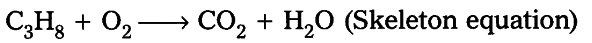Step 2 : Compare number of atoms of each element on both sides.

Find the coefficients to balance the equation. In this case, there are 3 carbon atoms on the left side of the equation but only one on the right side. If we add a coefficient of 3 to {{CO}_{2}} on the right side the carbon atoms balance.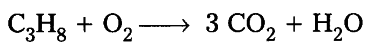Now, look at the number of hydrogen atoms. There are 8 hydrogen atoms on the left but only 2 on the right side. By adding a coefficient of 4 to the H_{2}O on the right side, the hydrogen atoms get balanced.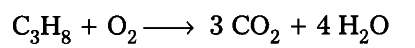Finally, look at the number of oxygen atoms. There are 2 on the left side but 10 on the right side. By adding a coefficient of 5 to the O_{2} on the left side, the oxygen atoms get balanced.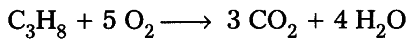Step 3 : Make sure the coefficients are reduced to their smallest whole number values. The above equation is already with the coefficients in smallest whole numbers. There is no need to reduce its coefficients. Hence the final equation is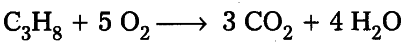Step 4 : Check the answer. Count the numbers and kinds of atoms on both sides of the equation to make sure they are the same.